# Computing Jerk in 2D Space

Hi everyone,

I am a graduate student in psychology and am working on a research project where I am trying to derive the kinematics of a mouse tracking task. I am currently writing a MATLAB script to do this, and I believe my equations for velocity and acceleration are correct, but I have very little background in physics/calculus and am having difficulty computing the 3rd derivative of position, jerk. I know this forum is for highschool/undergraduate level students, but I believe this question is too simple to post in the other forum.

1. Homework Statement

I have calculated the euclidean distances between all xy samples and determined the sum of the euclidean distances to determine displacement for the entire movement and the displacement between each pair of xy coordinates.

With displacement:
I have calculated the average velocity with the formula "sum of euclidean distance/total time of movement.

I have calculated the velocity profile using the the euclidean distances between each pair of xy coordinates divided by the sample rate (the time between each sample, so .5ms).
In regards to calculating acceleration, I have used the same formulas as above, but replaced displacement with velocity (the rate of change of velocity)

However, in regards to jerk (the rate of change of acceleration), I am trying to make sure I am calculating this correctly. I keep finding different formulas, but I am not certain all of them apply to 2D kinematics. If jerk is the rate of change of acceleration, am I wrong in thinking it can be calculated by acceleration divided by the time between each xy coordinate pairs?

I think my main issue is digesting the formulas. I have read quite a bit about calculating the derivatives of position, but with my limited understanding of physics, it is difficult to determine if the formulas are applicable to above mentioned 2D coordinate plane situation.

I really appreciate the any advice/help anyone can offer!

Best

Last edited by a moderator:

The only difference between 2D and "1D" Kinematics is you have x and y components. So anything that applies to 1 dimension should easily apply to 2 with the help of a little trig and geometry. What different formulas are your getting?

Hi,

Thank you for the reply! I am using the following formulas.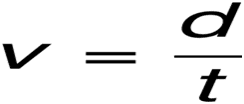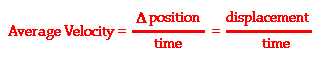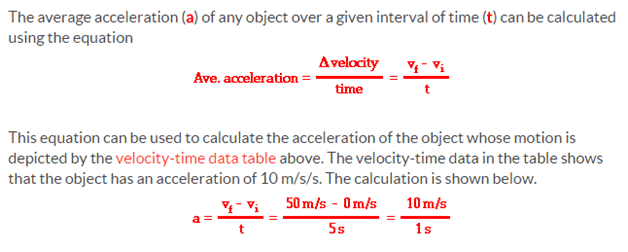Here are the jerk formulas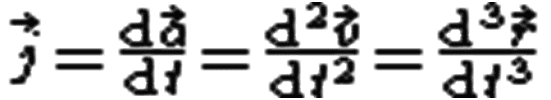I assume this is the same as above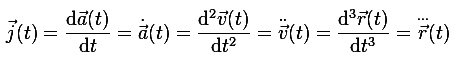Those are the formulas I have found. The rest of the confusion comes from journal articles talking about jerk of arm movements. I am not sure if that is applicable to my situation. Does it seem like my thinking about the variables is correct?

Thank you.

#### Attachments

haruspex
Homework Helper
Gold Member
Hi,

Thank you for the reply! I am using the following formulas.
View attachment 78416
View attachment 78417
View attachment 78418

Here are the jerk formulas
View attachment 78419
I assume this is the same as above
View attachment 78421
Those are the formulas I have found. The rest of the confusion comes from journal articles talking about jerk of arm movements. I am not sure if that is applicable to my situation. Does it seem like my thinking about the variables is correct?

Thank you.
That all looks ok, though I advise caution when dealing with real data. Each step in the differentiation chain can turn small errors into larger ones. You could apply some smoothing, but if overdone that will understate the values. You need to be aware of the precision of the original data and what that says about the answers.
You mention conflicting information regarding jerk of arms, but don't indicate what the conflict is. Is it possible those are not using "jerk" in this formal sense?